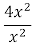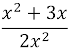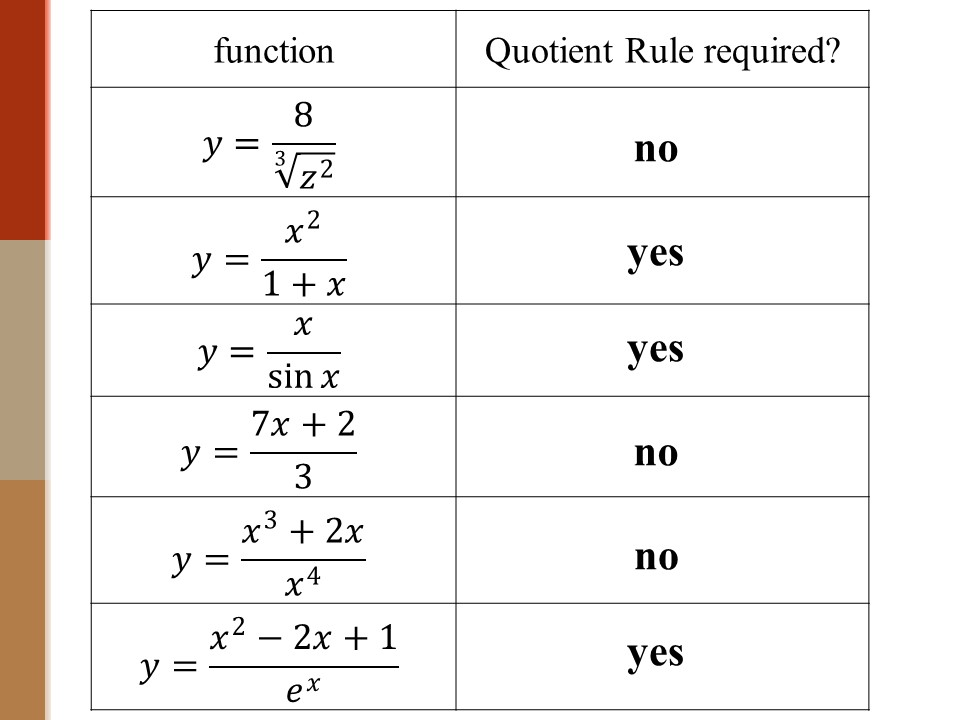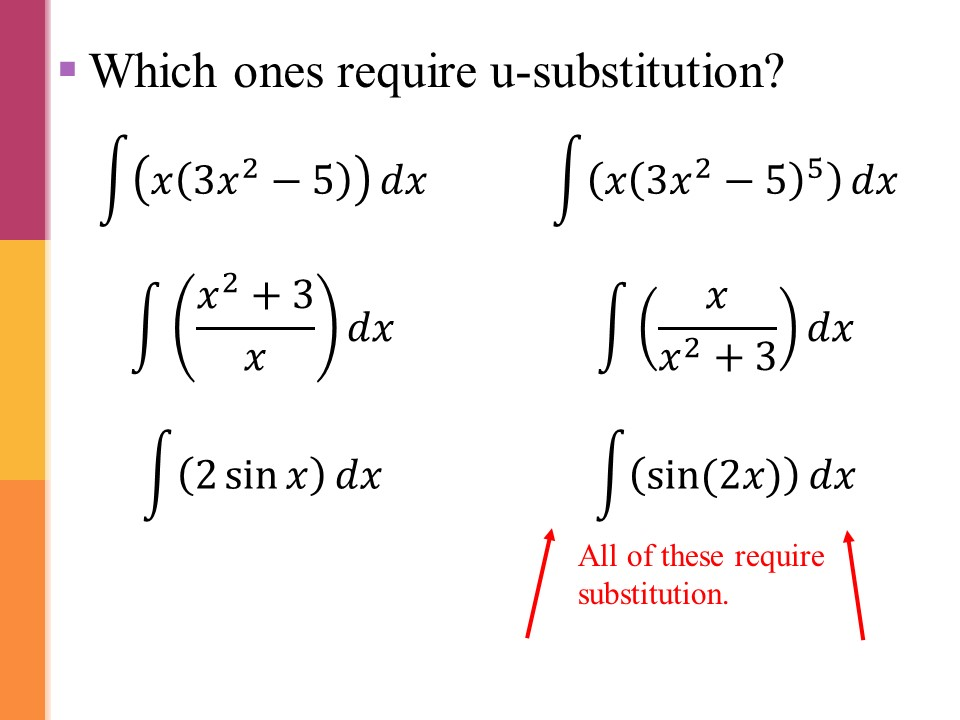•BLOG
•FREE STUFF
•SHOPHey there!
I've been teaching high school math for over 20 years. I write about my experiences here and also share activities that I have done with my classes that have been successful (and some that have been not so successful).
WANNA KNOW MORE?

# 12 Common Mistakes in Algebra and How to Fix Them

If you've been teaching high school math for a while, then you are familiar with common algebra mistakes that students make. In this post, I will address some of these errors and give suggestions on how to fix them (a.k.a. "the treatment").

The errors mentioned in this post are mistakes that I have seen from my Precalculus and Calculus students.

### 1) Incorrectly reducing rational expressions

Examples:

Diagnosis
• Students may not understand the definition of a factor.
• Students may be confusing “terms” with “factors.”
Treatment
• Teachers should avoid using phrases like “cancel” instead say “reduce to one” or “sum to zero.”
In expressions like this
x² + 3x – x²

Instead of saying, “x² and negative x² cancel,”

say, “x² and negative x² sum to zero.”

In expressions likeinstead of saying, “x² and x² cancel,”

say, “x² over x² reduces to 1.”

This should help students to avoid thinking they can “cancel” the x² in an expression like### 2) Not understanding what “like terms” are

Examples

x² + xx³

sinθ + sin²θ ≠ sin³θ

Diagnosis
• This is an example of students not knowing what are “like terms.”
Treatment
• Remind your students that if they are unsure if x² + x is x³, they should substitute a number for x. For example, let x = 3.
On the left: 3² + 3, which is 12.

On the right: 3³ is 27

Since 27 ≠ 12, then you know that x² + xx³.

### 3) Incorrectly expanding binomials

Example

Diagnosis
• This error stems from students learning this property of exponents: (ab)² = a²b², then thinking that one can “distribute” the exponent to every term in the parentheses.
Treatment
• Avoid using the word “distribute” when teaching properties of exponents.
• Remind students that exponents are a fast way to multiply, so (x + 2)² is the same thing as (x + 2)(x + 2)

### 4) Incorrectly taking roots

Example
• This error happens after students learn this property of radicals:
then thinking that one can always put the radical on each inside term.

Treatment
• Remind students of the definition of a square root. We know that
because if we square 3, we get 9.

Students can use this logic to check their answer by squaring their answer (e.g. x+2) to see if they get the expression under the radical (x² + 4).

### 5) Not using the Distributive Property correctly

Examples

Diagnosis
• Students either do not understand the Distributive Property or have forgotten to use it.
• In the second example, students often get confused by the negative because nothing is written between the negative sign and the open parenthesis.
Treatment
• Students need to be reminded of the Distributive Property.
• In the second example, show students how a 1 can be written just before the open parenthesis, which should help them see that they will distribute a negative one in the first step.

### 6) Not following the Order of Operations

Examples

Diagnosis
• Students want to do the first operation they see on the left and ignore the Order of Operations.
Treatment

### 7) Cross multiplying every time two fractions are next to each other

Example
6x = 35

Diagnosis
• Students who think they should cross multiply when multiplying fractions have forgotten about the Properties of Proportions.
Treatment
• My on-level calculus students always said “cross multiply” when I would ask them how to multiply fractions.
• I made a poster of the Properties of Proportions (available for FREE) to remind them about when (and why) we can cross multiply.

### 8) Not using the Zero Product Property

Example
x(x  1) = 2
x = 2 or x  1 = 2

Diagnosis
• Students have translated the Zero Product Property (ZPP) to mean “set each factor equal to whatever is on the other side of the equal sign.”
Treatment
• This is a frustrating error for me because I saw many of my on-level calculus students do this even though they had learned the ZPP years before.
• One way to overcome this error with students is to say the name of the property every time it is used – emphasizing the word “zero.”
• You could even remind students why the property works (if the product of two quantities is zero, of course, one of them must be zero) so they can then reason that it doesn’t work with other numbers (e.g., if the product of two quantities is 12, it does not mean that one of them must be 12.)
*Fun fact: I used to teach from an Australian textbook. They call this the Null Factor Law

### 9) Dividing by a variable or a function

Examples
x = 4x – x²
1 = 4 – x

sin x cos x = sin x
cos x = 1
Diagnosis
• Students think that since they can divide an entire equation by a constant, they can also divide an entire equation by a variable (or a function).
Treatment
• I tell students that this is illegal because they are dividing by an unknown quantity and could be dividing by zero.
• It can be difficult for students to realize they made a mistake in this process since they will likely be correct when checking their answers, but they will not have all the answers.
• As a teacher, you can train students to factor out common factors instead of dividing by them.
For example, 2+ 4 = 0

Instead of dividing by 2, you could factor out the 2 to get:

2(+ 2) = 0, then use the ZPP to get
2 = 0 or + 2 = 0
Obviously, 2 ≠ 0, so that can be disregarded.

### 10) Not understanding properties of logarithms

Example
log x = log x + log(x  4)
x = x + (x   4)
Diagnosis
• Students who make this mistake are not familiar enough with the Properties of Logarithms. They forget to apply the Product Property before applying the One-to-One Property.
Treatment
• Remind students of the Properties of Logarithms and ensure they get plenty of opportunities to practice before an assessment.
• Formative assessments (whose grades don’t go in the gradebook) are an excellent way to stop these errors before they cost students points. Read my blog post How to Give a Formative Assessment in Secondary Math

### 11) Not understanding function notation correctly

Examples

Diagnosis
• Students are not understanding function notation and think that sin x represents multiplication.
Treatment
• Always say the word "of" when talking about a function so that students can make the connection between f(x) and sin(x) or log(x).
• Usually, students work so much with f(x) that they learn early that f(2) ≠ 2f. Therefore, use that to help them understand that sin(2) does not mean multiplying sine times 2 (which is nonsense anyway). This should help them avoid a mistake like (x)(sin(x) ≠ sin(x²)
• Always write the parentheses around the x when using a trig function or log function to emphasize that the x is attached to the “sin” or “log.”

### 12) Not understanding how to undo operations with rational expressions:

Example

and this:

Treatment:
• Reinforce with the students that when “splitting a fraction,” they are just undoing either addition/subtraction or multiplication of fractions.
• Students should check their answers to determine whether they would get the given expression if they “operated” with their answer.

#### General suggestions for fixing errors

• Make an activity where students are given expressions and asked how to simplify each expression - they don't have to actually simplify them, just say *how* to simplify or if it can be simplified.
• If I am limited on time, then I will do it as a whole class activity. Below are some examples I have used in my calculus class:After going through the Quotient Rule for Differentiation examples, I went through this slide with my students.After doing u-substitution examples for integration, I went through this slide with my students.

#### In summary

To correct most of these procedural errors, students must become more familiar with the rules, properties, and theorems in mathematics. In other words, you've got to know the rules if you want to play the game!

Think of the first time you learned to play Monopoly or Uno. Probably someone was there to teach you. Perhaps that person read the rules and How to Play that came in the box. Or maybe someone just said, "the best way to learn to play is to play," and then taught you the rules as you went along.

Well, learning algebra is a bit like that. You have to know what the rules, properties, theorems, and constraints are before you can "play" algebra.